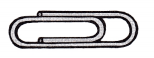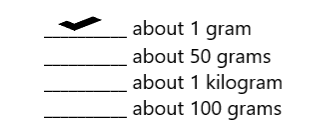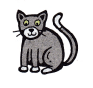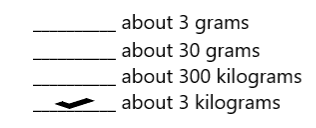# McGraw Hill Math Grade 4 Chapter 11 Lesson 1 Answer Key Metric Units of Mass

Practice the questions of McGraw Hill Math Grade 4 Answer Key PDF Chapter 11 Lesson 1 Metric Units of Mass to secure good marks & knowledge in the exams.

## McGraw-Hill Math Grade 4 Answer Key Chapter 11 Lesson 1 Metric Units of Mass

Solve.

Question 1.
What is the mass of a paperclip?Explanation:
The mass of a paperclip is about 1 gram.

Question 2.
What is the mass of a cat?Explanation:
The mass of a cat is about 3 kilograms.

Multiply to find each missing number.

Question 3.
1 kg = __________ g
1000g

Explanation:
1 kg is equal to 1000 grams.

Question 4.
4 kg = __________ g
4000 g

Explanation:
1 kg = 1000 g
2 kg = 2 x 1000 = 2000 g
So, 2 kg = 2000 g.

Question 5.
13 kg = __________ g
13000g

explanation:
1 kg = 1000 g
13 kg = 13 x 1000 = 13000 g
so, 13 kg = 13000 g.

Question 6.
57 kg = __________ g
57,000g

Explanation:
1 kg = 1000 g
57 kg = 57 x1000 = 57,000 g
so, 57 kg = 57,000 g.

Question 7.
472 kg = __________ g
472,000 g

Explanation:
1 kg = 1000 g
472 kg = 472 x 1000 = 472,000 g
so, 472 kg = 472,000 g.

Question 8.
397 kg = __________ g